# Compare different regression methods

## 1. The compare_methods()

This is a short vignette about the compare_methods() function from the dendroTools R package. The compare_methods() uses k-fold cross-validation to compare different regression methods. In addition, methods could be compared by 1) using a holdout data, where data is excluded from the hyperparameter optimization and cross-validation and methods are later additionally evaluated for those points, or 2) similarly by using edge data, where extreme (edge) points are estimated as a validation data. Currently, there are five regression methods implemented: artificial neural networks with the Bayesian regularization training algorithm (BRNN), (ensemble of) model trees (MT), random forests of regression trees (RF) and (multiple) linear regression (MLR). The calculated performance metrics are the correlation coefficient (r), the root mean squared error (RMSE), the root relative squared error (RRSE), the index of agreement (d), the reduction of error (RE), the coefficient of efficiency (CE), the detrended efficiency (DE) and mean bias, calculated as the difference between observed and estimated mean response for the validation and calibration data. The output of the compare_methods() function is a list with 18 elements, which could be retrieved by calling the “$” operator and the element name. Please note, the examples presented here are made computationally less intensive to satisfy the CRAN policy. Element Element_description$mean_std data frame with calculated metrics for the selected regression methods. For each regression method and each calculated metric, mean and standard deviation are given
$std_ranks data frame with ranks of calculated metrics: mean rank and share of rank_1 are given$edge_results data frame with calculated performance metrics for the central-edge test. The central part of the data represents the calibration data, while the edge data, i.e. extreme values, represent the validation data. Different regression models are calibrated using the central data and validated for the edge (extreme) data. This test is particularly important to assess the performance of models for the prediction of the extreme data. The share of the edge (extreme) data is defined with the edge_share argument
$holdout_results calculated metrics for the holdout data$bias_cal ggplot object of mean bias for calibration data
$bias_val ggplot object of mean bias for validation data$transfer_functions ggplot or plotly object with transfer functions of different methods, facet is used to separate methods
$transfer_functions_together ggplot or plotly object with transfer functions of methods plotted together$parameter_values a data frame with specifications of parameters used for different regression methods
$PCA_output princomp object: the result output of the PCA analysis$reconstructions ggplot object: reconstructed dependent variable based on the dataset_complete argument, facet is used to split plots by methods
$reconstructions_together ggplot object: reconstructed dependent variable based on the dataset_complete argument, all reconstructions are on the same plot$normal_QQ_cal normal q-q plot for calibration data
$normal_QQ_holdout normal q-q plot for holdout data$normal_QQ_edge normal q-q plot for edge data
$residuals_vs_fitted_cal residuals vs fitted values plot for calibration data$residuals_vs_fitted_holdout residuals vs fitted values plot for holdout data
$residuals_vs_fitted_edge residuals vs fitted values plot for edge data ## 2. Basic example For the basic example, we will use the dataset with the Mean Vessel Area (MVA) chronology and the mean April temperature, the dataset is saved as dataset_MVA. All five regression methods will be compared with 10-fold cross-validation repeated 1 times. The optimize argument is set to TRUE, therefore all tuning parameters will be defined in a preliminary optimization phase. After the comparison, the output elements are retrieved with the “$” operator.

# Load the dendroTools R package
library(dendroTools)

data(dataset_MVA)

# Basic example
basic_example <- compare_methods(formula = T_Apr ~ MVA, dataset = dataset_MVA, k = 10, repeats = 1, optimize = TRUE, MT_committees_vector = c(1), RF_maxnodes_vector = c(5), RF_nodesize_vector = c(10))
# The data frame with mean and standard deviation of performance metrics for the calibration and the validation data
kable(basic_example$mean_std) Metric Period Mean BRNN Std BRNN Mean MLR Std MLR Mean MT Std MT Mean RF Std RF 1 r cal 0.607 0.026 0.596 0.026 0.596 0.026 0.712 0.016 2 r val 0.632 0.286 0.635 0.278 0.635 0.278 0.567 0.321 3 RMSE cal 1.162 0.036 1.174 0.036 1.174 0.036 1.032 0.028 4 RMSE val 1.186 0.326 1.193 0.335 1.193 0.335 1.235 0.318 5 RRSE cal 0.794 0.020 0.802 0.020 0.802 0.020 0.706 0.017 6 RRSE val 0.879 0.219 0.884 0.221 0.884 0.221 0.921 0.244 7 d cal 0.716 0.024 0.709 0.025 0.709 0.025 0.791 0.017 8 d val 0.669 0.149 0.664 0.147 0.664 0.147 0.653 0.165 10 RE val 0.237 0.426 0.229 0.432 0.230 0.431 0.156 0.530 12 CE val 0.183 0.420 0.175 0.427 0.175 0.427 0.097 0.524 14 DE val -0.300 1.053 -0.305 1.027 -0.305 1.027 -0.500 1.600 # The data frame with non-parametric estimation of different methods: average rank and share of rank one kable(basic_example$rank)
Metric Period Avg rank BRNN %rank_1 BRNN Avg rank MLR %rank_1 MLR Avg rank MT %rank_1 MT Avg rank RF %rank_1 RF
13 r cal 2.0 0.0 3.3 0.0 3.6 0.0 1.0 1.0
14 r val 2.4 0.2 1.9 0.3 2.3 0.3 3.4 0.2
7 RMSE cal 2.0 0.0 3.0 0.0 4.0 0.0 1.0 1.0
8 RMSE val 2.3 0.3 2.1 0.4 2.3 0.1 3.3 0.2
9 RRSE cal 2.0 0.0 3.0 0.0 4.0 0.0 1.0 1.0
10 RRSE val 2.3 0.3 2.1 0.4 2.3 0.1 3.3 0.2
11 d cal 2.2 0.0 3.4 0.0 3.4 0.0 1.0 1.0
12 d val 2.1 0.3 2.6 0.1 2.4 0.3 2.9 0.3
6 RE val 2.3 0.3 2.1 0.4 2.3 0.1 3.3 0.2
2 CE val 2.3 0.3 2.1 0.4 2.3 0.1 3.3 0.2
4 DE val 2.3 0.3 2.1 0.4 2.3 0.1 3.3 0.2
# See the histogram of mean bias for the validation data
basic_example$bias_val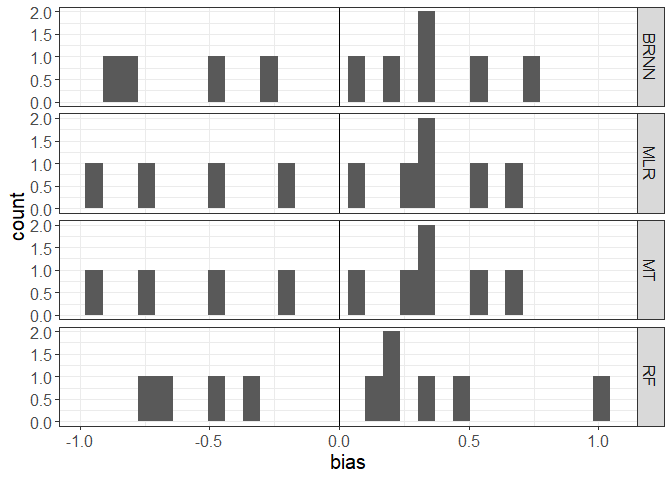Histogram for the validation data for the basic_example # See the histogram of mean bias for the calibration data basic_example$bias_cal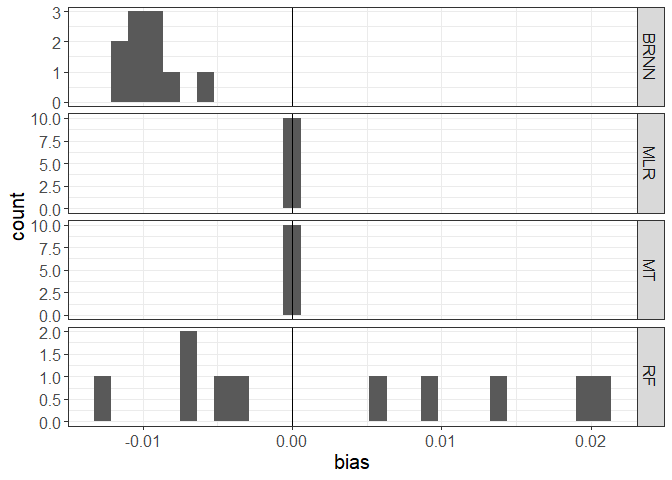Histogram for the calibration data for the basic_example

# See the transfer functions, separated by facets. This is a ggplot object and could be easily customized.
library(ggplot2)
basic_example$transfer_functions + xlab(expression(paste('MVA [',mm^2,']'))) + ylab("April Mean Temperature [°C]")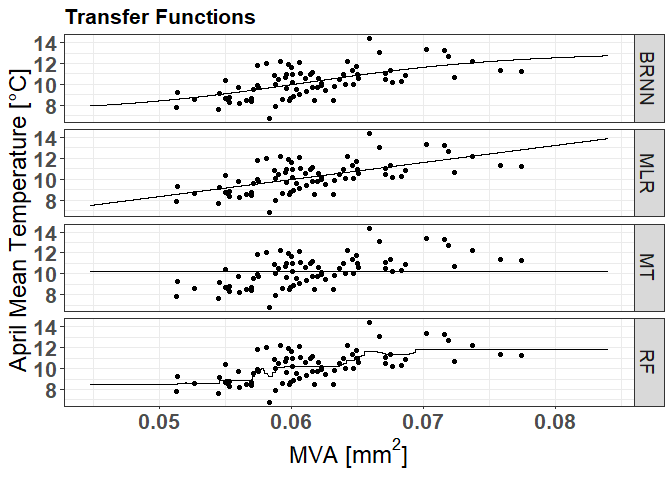The transfer functions of different methods, facet is used to separate plots by method. # See the transfer functions, plotted together. This is a ggplot object and could be easily customized. basic_example$transfer_functions_together +
xlab(expression(paste('MVA [',mm^2,']'))) +
ylab("April Mean Temperature [°C]")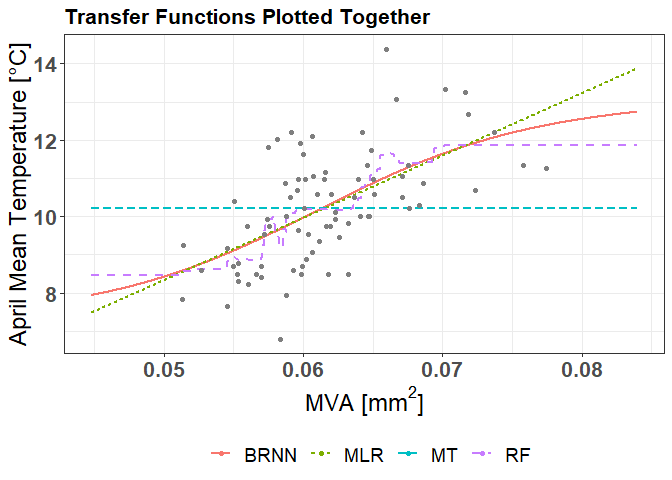The transfer functions of different methods, all functions are on the same plot, therefore it is easy to see the differences among different methods.

# The data frame of optimized tuning parameters for different methods
kable(basic_example$parameter_values) Method Parameter Considered values Selected value BRNN BRNN_neurons 1, 2, 3 2 MT MT_committees 1 1 MT MT_neighbors 0, 5 5 MT MT_rules 100, 200 200 MT MT_unbiased TRUE, FALSE TRUE MT MT_extrapolation 100 100 MT MT_sample 0 0 RF RF_mtry 1 1 RF RF_maxnodes 5 5 RF RF_ntree 100 250 500 250 RF RF_nodesize 10 10 # For calibration data, there are residual diagnosti plots available. Similar plots are available also for holdout and edge data. library(gridExtra) grid.arrange(basic_example$normal_QQ_cal, basic_example$residuals_vs_fitted_cal, ncol = 2) ## geom_smooth() using formula 'y ~ x'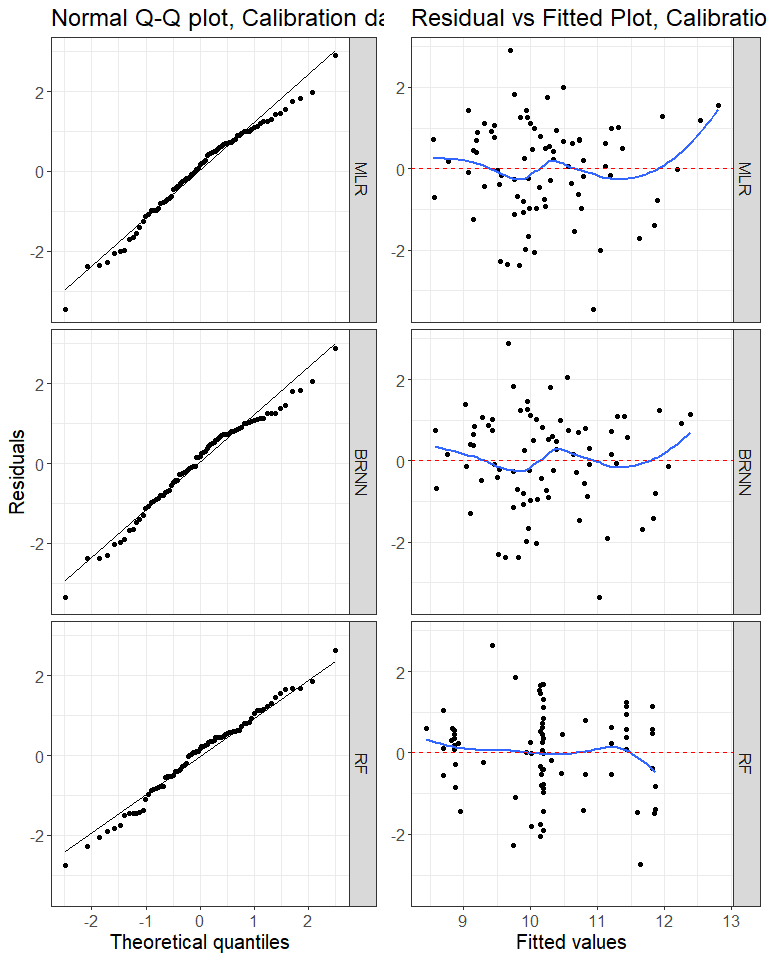Residual diagnostic plots for calibration data: Normal Q-Q plot (left plot) and residuals vs fitted plot (right plot) ## 3. Principal component analysis in combination with the compare_methods() Principal Component Analysis (PCA) is commonly used with tree-ring data to reduce the full set of original tree-ring chronologies to a more manageable set of transformed variables. These transformed variables, the set of principal component scores, are then used as predictors in climate reconstruction models. The PCA also acts to strengthen the common regional-scale climate response within a group of tree-ring chronologies by concentrating the common signal in the components with the largest eigenvalues. To use PCA regression within the compare_methods(), set the argument PCA_transformation to TRUE. All independent variables in the dataset data frame will be transformed using the PCA transformation. If the parameter log_preprocess is set to TRUE, variables will be transformed with logarithmic transformation before used in PCA. With the argument components_selection, we specify how to select PC scores that will be used as predictors. There are three options: “automatic”, “manual” and “plot_selection”. If argument is set to “automatic”, all PC scores with eigenvalues above 1 will be selected. This threshold could be changed by changing the eigenvalues_threshold argument. If argument is set to “manual”, user should set the number of components with N_components argument. If components_selection is set to “plot_selection”, A scree plot will be shown, and a user must manually enter the number of components to be used as predictors. The latter seems to be the most reasonable choice. For the example with PCA, we use dataset dataset_MVA_individual, which consist of 10 individual Mean Vessel Area (MVA) chronologies and mean April temperature for the Ljubljana region, Slovenia. The dataset has 56 observations. The selection of components is set to “manual”, N_components to be used in the later analysis is set to 2. In this example, the 5-fold cross-validation with 1 repeat will be used to compare MT, MLR and BRNN. The subset of methods could be set with the methods argument. The argument optimize is set to TRUE, therefore all tuning parameters will be set automatically. # Load the dendroTools R package library(dendroTools) # Load data data(dataset_MVA_individual) # Example PCA example_PCA <- compare_methods(formula = T_Apr ~ ., dataset = dataset_MVA_individual, k = 5, repeats = 1, optimize = TRUE, methods = c("MLR", "MT", "BRNN"), PCA_transformation = TRUE, components_selection = "manual", N_components = 2, seed_factor = 5, MT_committees_vector = c(1), RF_maxnodes_vector = c(5), RF_nodesize_vector = c(10)) # Get the summary statistics for the PCA summary(example_PCA$PCA_output)
## Importance of components:
##                           Comp.1    Comp.2    Comp.3     Comp.4     Comp.5
## Standard deviation     1.8691406 1.5007125 1.1867201 0.87168877 0.79432916
## Proportion of Variance 0.3493686 0.2252138 0.1408305 0.07598413 0.06309588
## Cumulative Proportion  0.3493686 0.5745824 0.7154129 0.79139704 0.85449292
##                            Comp.6     Comp.7     Comp.8     Comp.9     Comp.10
## Standard deviation     0.68818504 0.61467322 0.57233447 0.49611738 0.173060035
## Proportion of Variance 0.04735987 0.03778232 0.03275667 0.02461325 0.002994978
## Cumulative Proportion  0.90185279 0.93963510 0.97239178 0.99700502 1.000000000
# The mean and standard deviation data frame
kable(example_PCA$mean_std) Metric Period Mean BRNN Std BRNN Mean MLR Std MLR Mean MT Std MT 1 r cal 0.646 0.025 0.639 0.024 0.631 0.024 2 r val 0.543 0.227 0.542 0.208 0.467 0.315 3 RMSE cal 1.157 0.068 1.164 0.070 1.176 0.083 4 RMSE val 1.234 0.286 1.245 0.288 1.304 0.259 5 RRSE cal 0.764 0.021 0.768 0.020 0.775 0.020 6 RRSE val 0.971 0.247 0.975 0.226 1.043 0.318 7 d cal 0.743 0.022 0.745 0.020 0.738 0.020 8 d val 0.663 0.114 0.666 0.107 0.628 0.157 10 RE val 0.286 0.254 0.282 0.214 0.174 0.371 12 CE val 0.009 0.490 0.009 0.447 -0.169 0.690 14 DE val -0.178 0.692 -0.177 0.657 -0.397 0.941 ## 4. Example of multiproxy analysis The compare_methods() enables the comparison of methods for regression problems with two or more independent variables. However, users should select multiple proxies reasonably and with caution, since there is nothing to prevent from including colinear variables. To perform the comparison of methods with multiproxy variables, simply include dataset with more than one independent variable and specify the relationship with the formula argument. If metrics on validation data are much lower than on calibration data, there is a problem of overfitting and users should exclude some independent variables and repeat the analysis. For the multiproxy_example, we will use example_dataset_1, which consist of the Mean Vessel Area (MVA) chronology and two temperature variables, the mean April temperature (T_APR) and the mean temperature from August to September (T_aug_sep) from the previous growing season. To compare methods with multiproxy approach, specify formula with two independent variables, such as formula = MVA ~ T_APR + T_aug_sep. Here, we will compare MT, BRNN and RF with 10-fold cross-validation and 1 repeat. # Load the dendroTools R package library(dendroTools) # Load data data(example_dataset_1) # Example multiproxy example_multiproxy <- compare_methods(formula = MVA ~ T_APR + T_aug_sep, dataset = example_dataset_1, k = 10, repeats = 1, optimize = FALSE, methods = c("MT", "BRNN", "RF")) # The mean and standard deviation data frame kable(example_multiproxy$mean_std)
Metric Period Mean BRNN Std BRNN Mean MT Std MT Mean RF Std RF
1 r cal 0.723 0.018 0.693 0.036 0.809 0.014
2 r val 0.690 0.243 0.639 0.290 0.634 0.234
3 RMSE cal 0.405 0.013 0.422 0.018 0.362 0.013
4 RMSE val 0.409 0.112 0.441 0.138 0.449 0.098
5 RRSE cal 0.690 0.019 0.719 0.034 0.618 0.017
6 RRSE val 0.792 0.325 0.847 0.343 0.860 0.273
7 d cal 0.817 0.015 0.795 0.031 0.845 0.011
8 d val 0.729 0.186 0.685 0.204 0.660 0.152
10 RE val 0.326 0.694 0.231 0.751 0.253 0.619
12 CE val 0.277 0.704 0.176 0.761 0.193 0.623
14 DE val 0.026 0.918 -0.101 0.998 -0.149 0.845

## 5. Example of climate reconstruction

Reconstructions of past climate conditions include reconstructions of past temperature, precipitation, vegetation, streamflow, sea surface temperature, and other climatic or climate-dependent conditions. With the compare_methods() it is possible to directly reconstruct the dependent variable specified with the formula argument. To do so, supply additional complete dataset with tree-ring chronology that goes beyond the observations of instrumental records.

For the example_reconstruction, we use data_TRW dataset, which includes a tree-ring width (TRW) chronology of Pinus nigra from Albania and mean June-July temperature from Albania. The complete TRW chronology is supplied with the dataset_TRW_complete. In this example, we will compare RF and MLR models with 3-fold cross-validation and 1 repeat.

# Load the dendroTools R package
library(dendroTools)

data(dataset_TRW)
data(dataset_TRW_complete)

# Example reconstruction
example_reconstruction <- compare_methods(formula = T_Jun_Jul ~ TRW, dataset = dataset_TRW, k = 3, optimize = FALSE, methods = c("MLR", "BRNN", "MT", "RF"), dataset_complete = dataset_TRW_complete)
example_reconstruction$reconstructions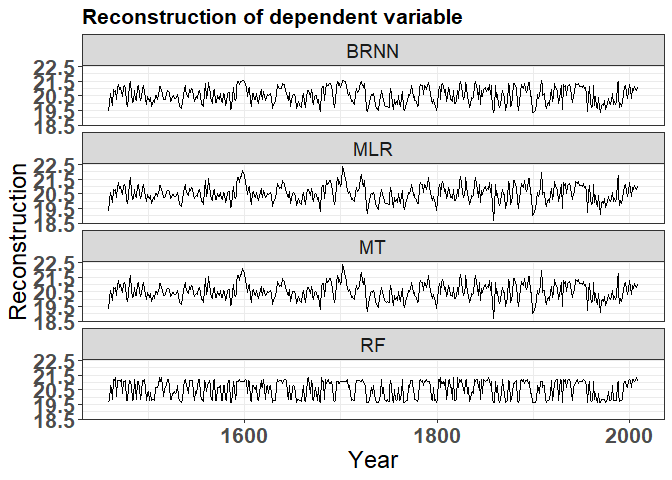The reconstructed June-July temperatures based on the dataset_complete argument, facet is used to split plots by methods. example_reconstruction$reconstructions_together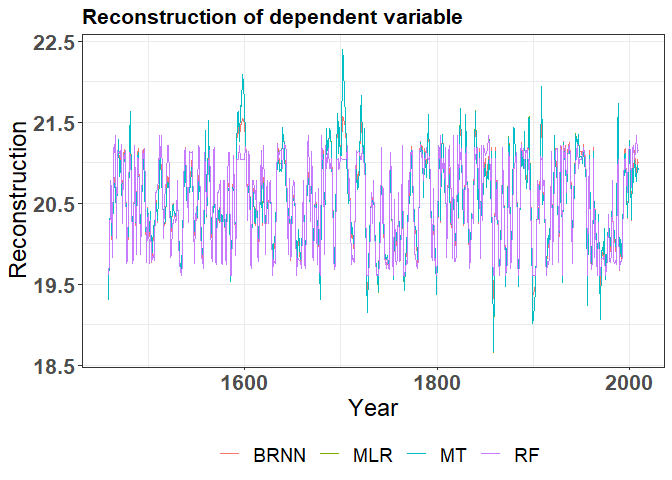The reconstructed June-July temperatures based on the dataset_complete argument, all reconstructions are on the same plot. The RF model reconstructed temperatures with much lower variance than the MLR model.

The comparison among different methods usually shows, that different regression techniques perform relatively similar for the central-calibration data, while for the extreme values, predictions differ. Therefore, we implemented additional central-edge test, where the central part of the dataset represents the calibration data, while the edge data, i.e. extreme values, represent the edge data. Different regression models are calibrated using the central data and validated using the edge (extreme) data. This test is particularly important to assess the performance of different methods for the prediction of the extreme and out of calibration data. The share of the edge (extreme) data is defined with the edge_share argument. To get the results for the central-edge test, use example_reconstruction$edge_results. # The central-edge test kable(example_reconstruction$edge_results)
Metric Period BRNN MLR MT RF
r cal_central 0.648 0.645 0.645 0.775
r val_edge 0.756 0.749 0.749 0.761
RMSE cal_central 0.687 0.689 0.689 0.576
RMSE val_edge 0.752 0.918 0.918 0.606
RRSE cal_central 0.762 0.764 0.764 0.639
RRSE val_edge 0.822 1.003 1.003 0.663
d cal_central 0.750 0.751 0.751 0.841
d val_edge 0.861 0.831 0.831 0.874
RE val_edge 0.326 -0.004 -0.003 0.562
CE val_edge 0.324 -0.006 -0.006 0.561
DE val_edge -0.066 -0.588 -0.588 0.307

In this example, MLR and MT had worse validation results than RF and BRNN. This indicates that linear interpolation for extreme values is not the best choice.

## 6. Tuning the machine learning parameters

Machine learning methods have several tuning parameters that need to be set, e.g. the number of neurons for the BRNN (parameter BRNN_neurons = 2). By default the optimize argument is set to TRUE, therefore all parameters will be automatically optimized in a preliminary cross-validation phase, where different combinations of parameters are tested and the best combination for each method is later used for the final model comparison. Each parameter has a pre-defined vector of possible values, however, this vector of possible values could be extended and therefore a wider space of tuned values could be explored. To change the vector of possible values for the BRNN_neurons parameter, use e.g. BRNN_neurons_vector = c(1, 2, 3, 4, 5). Bellow, see the table of all tuning parameters together with the vectors of possible values.

Method Parameter Vector_for_optimization
BRNN BRNN_neurons BRNN_neurons_vector
MT MT_committees MT_committees_vector
MT MT_neighbors MT_neighbors_vector
MT MT_rules MT_rules_vector
MT MT_unbiased MT_unbiased_vector
MT MT_extrapolation MT_extrapolation_vector
MT MT_sample MT_sample_vector
RF RF_mtry RF_mtry_vector
RF RF_maxnodes RF_maxnodes_vector
RF RF_ntree RF_ntree_vector
RF RF_nodesize RF_nodesize_vector

To set the tuning parameters manually, set the parameter optimize to FALSE and supply the selected value of each tuning parameter with the corresponding argument (if not, the default value will be used). Here is a simple example, where tuning parameters are set manually.

# Load the dendroTools R package
library(dendroTools)

data(example_dataset_1)

example_optimize <- compare_methods(formula = MVA ~  T_APR, dataset = example_dataset_1, k = 5, repeats = 2, optimize = FALSE, BRNN_neurons = 1, MT_committees = 1, MT_neighbors = 0, MT_rules = 100, MT_unbiased = FALSE, MT_extrapolation = 100, MT_sample = 0, RF_mtry = 1, RF_ntree = 100, RF_maxnodes = 20, seed_factor = 5)
# The data frame of tuning parameters, as defined by the user
kable(example_optimize\$parameter_values)
Method Parameter Selected value
BRNN BRNN_neurons 1
MT MT_committees 1
MT MT_neighbors 0
MT MT_rules 100
MT MT_unbiased FALSE
MT MT_extrapolation 100
MT MT_sample 0
RF RF_mtry 1
RF RF_maxnodes 20
RF RF_ntree 100
RF RF_nodesize 1